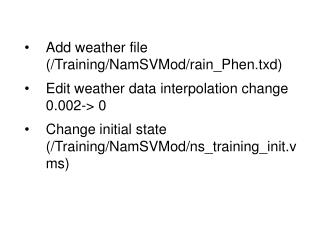DownloadDownload PresentationTélécharger la présentation- - - - - - - - - - - - - - - - - - - - - - - - - - - E N D - - - - - - - - - - - - - - - - - - - - - - - - - - -
##### Presentation Transcript

1. Add weather file (/Training/NamSVMod/rain_Phen.txd) Edit weather data interpolation change 0.002-> 0 Change initial state (/Training/NamSVMod/ns_training_init.vms)

2. Change pet calculation method to pan evaporation • Add timeseries point (Nam Oon Dam) • Add measurement file to the new timeseries point (/Training/NamSVMod/hq_NamOonDam.vms)

3. Choose timeseries output variables • Check computational parameters • Run model

4. Timeseries results, view qriver and precipitation results on Ban Tha Kok Daeng • Flow comparison results, Ban Tha Kok Daeng • Tools in Results window • saving: as picture and as values • One timeseries: grouping statistics (average), histogram • Two timeseries: R2 of common points, x-y plot • Selected timeseries: statistics, correlations • Editing pictures, change from line to dot, add header • bring together two timeseries • Results/Timeseries, results as table • Save project

5. Exercise 1 Compare the results from two different years at Ban Tha Kok Daeng (67,108) and Ts2 (138,80) + compare the measured and simulated flow at Ban Tha Kok Daeng • Use years 1992 and 1993 • Compare the following parameters: • qriver • prec • Copy the result to word

6. Exercise 2 Simulate the difference in flow if the precipitation increases 10% and evaporation increases 5% • Use year 1992 • Compare the following parameters: • qriver • prec • etr • Copy the result to word

7. Task 1 – sensitive analysis test • Objective: to understand the impact of different parameters on the river flow • Task: do sensitive analysis for following parameters: • Rainmult • Petcorr • Dz0 • Mnover use the ns_training.vmp as a basis. First calculate the model as it is for year 1992. Then increase the first parameter by 10% and compare to the base-result (average discharge at Ban Tha Kok Daeng). Then decrease the parameter by 10% based on the initial value and compare. Return the value for its original value. Repeat this to selected parameters • Result: analysis of the sensitive of four parameters and their impact on the Q

8. Task 2 – deforestration • Objective: to understand the impact of landuses on the model results • Task: analyse how much the landuse change impacts on the model results. Use years 1990-1993. First run the model as it is and save the results at two stations: • Ban Tha Kok Daeng (67,108) • Ts2 (138,80) then change all the landuse parameters from agriculture and irrigated agriculture to evergreen forest parameters (copy the parameters from the evergreen forest to agriculture and irrigated agriculture) • Result: calculate the change in average flow at selected two stations and plot the graph where those two model run are compared

9. Task 3 – climate change • Objective: to understand the impact of changes of precipitation and evaporation on the model results • Task: analyse how much the climate change impact on the model results. Use years 1990-1993. First run the model as it is and save the results at two stations: • Ban Tha Kok Daeng (67,108) • Ts2 (138,80) and save the results. Then increase the precipation 15 % and evaporation 7 % (change the rainmult and petcorr parameters in Landuse parameters... Menu). Compare the results • Result: calculate the change in average flow at selected two stations and plot the graph where those two model run are compared

10. Returning the tasks • Put all the results to one Word-document and send to Matti: matti.kummu@iki.fi by mid-June • Any questions, write to Matti • From www.eia.fi/wup-fin you can download the manuals • Next training planned for June, then the objective is 3D model including sediment and erosion modelling